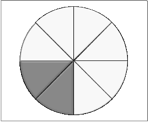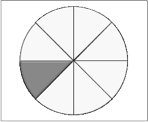# Conduct a Lesson Study Cycle on Fractions

## Prepare for Unit and Lesson Planning

In this module, your team will agree on the flow of the fractions unit and plan one lesson within the unit in depth, to serve as a research lesson. The research lesson will be taught by one team member to their students while other team members collect data on student responses, to be examined and discussed during the post-lesson discussion.

Closely examining lessons developed by others is an excellent way to dive into the Lesson Study process and to build the teaching profession. If you are intrigued by the potential of linear measurement context to introduce fractions or to build understanding of equivalence, you may want to use the video and materials from Modules 4 and 5 as the starting point for your research lesson. Or perhaps you would like to use insights about fractions gained from your work thus far to reshape your own curriculum’s lessons. In either case, this is a good time to:

Review Standards. We recommend that you revisit the Common Core State Standards [link downloadable fractions standards and whole standards] or your state standards as you begin planning for your research lesson. The Standards should help you identify aspects of student understanding you want to work on. Your state may also have a Progressions document related to your standards.

Revisit Your Insights From Your Team’s Work So Far. What have you learned so far about challenges in understanding fractions? Are there instructional tasks or approaches you are eager to try out with your students? Has your investigation so far illuminated elements of your instruction that you would like to work on?

The table below is an expanded version of the table first encountered in Module 2. It includes, in the right-hand column, some new ideas about how the linear measurement context might support students in overcoming key challenges in understanding fractions. Reviewing this table may help you look back on your work so far and finalize the design of your unit and research lesson within it.

 Type of Understanding or Knowledge Example of Student Difficulty or Understanding How Might a Linear Measurement Context Help? A Fraction is a Number A fraction represents an amount, not just pieces (such as 2 of 3 pieces of a pizza) or a situation (such as 2 of 3 shirts are red). When asked to put the fraction 2/3  on a number line, a student said “you can’t put it on a number line, because it’s two pieces out of three pieces, it’s not a number.” Or “ 2/3 is not a number, it’s two numbers.” [*] Linear measurement may help students shift from thinking “how many pieces” (counting) to thinking “how much” or “how long” (relative size). Students can partition a whole themselves and check whether the parts are equal; it is relatively easy to compare whether 2 lengths are equal, and to notice the relative size of part/whole. Fractions Can Be Greater than One May be difficult for students who have a strong image of a fraction as a piece of something. “You can’t have 6/5 because there’s only 5/5 in a whole.” When students measure an object that is longer than 1 foot (meter, etc.), it may be relatively easy to visualize something as a whole plus an additional fractional part and understand the meaning of fractions greater than 1. Fractions Can Be Partitioned A whole can be divided into smaller and smaller equal parts. The same fractional quantity can be represented by different fractions. Difficulty seeing how to divide a whole into equal parts. Difficulty seeing that 1/2 is equal to 2/4, 3/6, 4/8, 5/10 and so on. A number line (or ruler) may make it easy to see that the same point can be described by different fractions. What the Denominator Means Different units (such as 1/3  and 1/5) are different sizes. The more units a whole is partitioned into the smaller each one is. 1/n fits exactly n times into the whole. Students add 1/3 + 1/5 and get 2/8, without realizing they are adding two different things (thirds and fifths) sort of like adding apples and hammers. Students may think “1/5 is bigger than 1/4 because 5 is bigger than 4.” Difficulty seeing that 1/3 fits in the whole 3 times, 1/4 fits in the whole 4 times.  Has trouble seeing that 3/3, 4/4 etc. equal 1. Compared to fractional parts of area (which can be rearranged in many ways), length may provide a clear image of what is 1/3m, 1/2m, 10/11m, etc., and their relative size. Linear measurement may help provide a strong image that the unit that fits in 3 times is longer than the one that fits in 4 times, that 1/6 is half the length of 1/3, etc. Knowing What is the Whole Constructing the whole when given a fractional part. Keeping track of the whole. Difficulty making the whole when you give them a fractional part, e.g.: “This paper is 2/3; show me the whole.” Sees that the magnitude of a fraction depends on the magnitude of the whole (e.g., half of a small cookie is not the same as half of a large cookie) Confusion about whether the two drawings below together represent 3/8 of a pie or 3/16 of a pie.Using a standard measurement unit may be clearer, more familiar, and more stable than an ad hoc unit (such as pie pieces), making it easier to keep track of the whole. Fraction Size Understands that fraction size is determined by the (multiplicative) relationship between numerator and denominator – not just by the numerator, not just by the denominator, and not by the difference between numerator and denominator. Sees non-unit fraction as an accumulation of unit fractions. [A unit fraction has a numerator of 1; a non-unit fraction has a numerator other than 1.] May think 4/9 is bigger than 3/4 because 4 is bigger than 3 (comparing numerators), or 4/9 is bigger than 3/4 because 9 is bigger than 4 (comparing denominators), or 3/5 is the same size as 5/7 because the difference between the top and the bottom in both fractions is 2. Sees that equivalent fractions have the same multiplicative relationship between numerator and denominator. In 2/4, 4/8, 3/6, etc. denominator is two times numerator. Sees 5/8 is made up of five 1/8‘s or 5 times 1/8, that 9/8 is made up of 9 eighths or 9 times 1/8, etc. Length measurement may transfer to the number line more easily than some other models, so that students see the relative size of fractions. A familiar standard measurement unit (a meter, foot, etc.) may make it easy to see 1/3 as a length that goes in 3 times, 1/4 as a length that goes in 4 times, etc. When students think about a turtle who travels in a straight line 1/5 mile a day for 4 days, they may easily develop an image of 4/5 as four fifths—as 1/5 repeated four times along a number line.

Consider where your students are mathematically. If the students who will take part in the research lesson have not yet had an in-depth introduction to fractions, your team might want to introduce fractions using a linear measurement context, drawing on the grade 3 fractions lessons from the Japanese curriculum as replacement lessons.

If your students are in grade 4 or 5, the grade 3 Japanese lessons may still provide a good starting point from which you can go on to build understanding of equivalent fractions and the other grade 4 and 5 standards. Or you can use your work so far to consider the key understandings you want to build using your own curriculum.

Write the “Rationale” section of the Teaching-Learning Plan by briefly summarizing your current thoughts on what you want your research lesson to accomplish. Don’t worry about writing a perfect lesson rationale. The point is to consolidate your thoughts before you begin detailed planning of the lesson. You can revise your lesson rationale later.

Review the chart “Lesson Flow: Teaching Through Problem-Solving” below. When you watched the fractions lessons on video, you probably noticed that students built new mathematical understandings by solving and discussing a challenging problem, and that the new mathematical ideas were drawn from discussion of the students’ own work. The chart Lesson Flow: Teaching through Problem-Solving summarizes the flow of a Japanese teaching through problem-solving lesson, which is very similar to the lesson flow recommended in 5 Practices for Orchestrating Productive Mathematical Discussions, by Margaret S. Smith and Mary Kay Stein. Lesson study is an ideal opportunity to work with colleagues on a lesson structure that challenges students to develop as mathematical thinkers and problem-solvers.

#### Lesson Flow: Teaching Through Problem-Solving

 Lesson Phase Activity and Purpose Introduction and Posing the Task (brief) Teacher poses the problem. Students grasp it, become interested in solving it, and recall related ideas. Independent Problem-Solving  (7-20 min) Students bring their own prior knowledge to bear, trying to solve the problem. There may be input from classmates after students work for a few minutes on their own, but students are individually exerting effort to come up with a solution approach. Students are not simply following the teacher’s solution. Teacher circulates, noting student solution methods on a seating chart for teacher’s reference during next phase of the lesson. Teacher may question some students (e.g., “What is the problem asking?” to struggling students; “Can you write an equation to go with your diagram?” to students who think they are finished). Presentation and Class Discussion of Students’ Solution Approaches; this phase is orchestrated by teacher’s neriage (“kneading” or “polishing” discussion) (15-30 min) Teacher designates several students to present their work on the blackboard and explain it. Choice and sequence of the student work is planned by teacher in order to support development of the important mathematical understandings. (Incorrect approaches are sometimes included in the presentations.) Class members actively study the solutions, supported by teacher questions such as “How many solved it this way?” and “Do you agree with this method?” Students contrast solutions, supported by teacher questions, such as “What is the same and different about Sam’s and Marika’s solutions?” and “What are the good points and difficulties of each solution method?” Discussion focuses on the thinking and reasoning used in problem solving and the central mathematical ideas. Lesson Summary and Consolidation of Knowledge; may include assessment task (brief) Teacher draws on student thinking to summarize what has been learned (usually on blackboard). Students use the blackboard record and math journals to organize, reflect on, and consolidate their thinking. Class often ends with a journal writing prompt such as “What I learned today.”

## Develop the Teaching-Learning Plan

The Teaching-Learning Plan for a research lesson differs from an ordinary lesson plan because it is designed to stimulate, capture, and share your group’s learning, as well as to support the lesson instructor. The plan represents the thinking of the whole group about three concentric layers of practice—the lesson itself, the larger unit and subject area of which it is part, and the even larger domain of students’ long-term development of qualities like sense-making and persistence. As you move from planning to teaching the research lesson, your Teaching-Learning Plan will:

•      Support the lesson instructor by providing a detailed outline of the lesson and its logistical details (e.g., time, materials, wording of key questions);
•      Help your group anticipate student thinking, including misconceptions, and plan how student responses will be used to build learning within the lesson;
•      Help observers understand why your lesson is designed as it is, and what you hope to accomplish by teaching it;
•      Guide observers on data they should collect;
•      Provide forms to support careful observation, such as a student seating chart, Lesson Observation Notes (found at the end of Section 5), and a copy of the student task(s); and